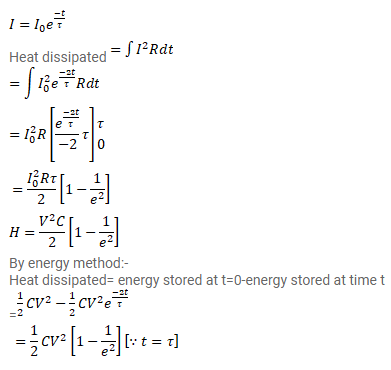# A capacitance C charged to a potential difference V is

Question:

A capacitance $\mathrm{C}$ charged to a potential difference $\mathrm{V}$ is discharged by connecting its plates through a resistance $\mathrm{R}$. Find the heat dissipated in one time constant after the connections are made. Do this by calculating $\int i^{2} R d t$ and also by finding the decrease in the energy stored in capacitor.

Solution: